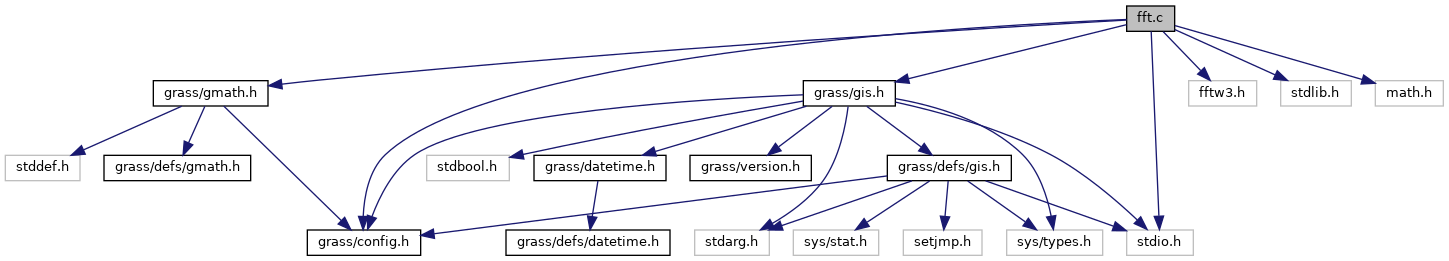GRASS GIS 7 Programmer's Manual  7.9.dev(2021)-e5379bbd7
fft.c File Reference

Fast Fourier Transformation of Two Dimensional Satellite Data functions. More...

`#include <grass/config.h>`
`#include <fftw3.h>`
`#include <stdlib.h>`
`#include <stdio.h>`
`#include <math.h>`
`#include <grass/gmath.h>`
`#include <grass/gis.h>`
Include dependency graph for fft.c:Go to the source code of this file.

## Macros

#define c_re(c)   ((c))

#define c_im(c)   ((c))

## Functions

int fft2 (int i_sign, double(*data), int NN, int dimc, int dimr)
Fast Fourier Transform for two-dimensional array. More...

int fft (int i_sign, double *DATA, int NN, int dimc, int dimr)
Fast Fourier Transform for two-dimensional array. More...

## Detailed Description

Fast Fourier Transformation of Two Dimensional Satellite Data functions.

This program is free software; you can redistribute it and/or modify it under the terms of the GNU General Public License as published by the Free Software Foundation; either version 2 of the License, or (at your option) any later version.

This program is distributed in the hope that it will be useful, but WITHOUT ANY WARRANTY; without even the implied warranty of MERCHANTABILITY or FITNESS FOR A PARTICULAR PURPOSE. See the GNU General Public License for more details.

You should have received a copy of the GNU General Public License along with this program; if not, write to the Free Software Foundation, Inc., 51 Franklin Street, Fifth Floor, Boston, MA 02110-1301 USA

Date
2001-2006

Definition in file fft.c.

## ◆ c_im

 #define c_im ( c ) ((c))

Definition at line 41 of file fft.c.

## ◆ c_re

 #define c_re ( c ) ((c))

Definition at line 40 of file fft.c.

## ◆ fft()

 int fft ( int i_sign, double * DATA, int NN, int dimc, int dimr )

Fast Fourier Transform for two-dimensional array.

Fast Fourier Transform for two-dimensional array.
<bNote: If passing real data to fft() forward transform (especially when using fft() in a loop), explicitly (re-)initialize the imaginary part to zero (DATA[i] = 0.0). Returns 0.

Parameters
 [in] i_sign Direction of transform -1 is normal, +1 is inverse [in,out] DATA Pointer to complex linear array in row major order containing data and result [in] NN Value of DATA dimension (dimc * dimr) [in] dimc Value of image column dimension (max power of 2) [in] dimr Value of image row dimension (max power of 2)
Returns
int always returns 0

Definition at line 127 of file fft.c.

Referenced by del2g().

## ◆ fft2()

 int fft2 ( int i_sign, double(*) data, int NN, int dimc, int dimr )

Fast Fourier Transform for two-dimensional array.

Fast Fourier Transform for two-dimensional array.
<bNote: If passing real data to fft() forward transform (especially when using fft() in a loop), explicitly (re-)initialize the imaginary part to zero (DATA[i] = 0.0). Returns 0.

Parameters
 [in] i_sign Direction of transform -1 is normal, +1 is inverse [in,out] data Pointer to complex linear array in row major order containing data and result [in] NN Value of DATA dimension (dimc * dimr) [in] dimc Value of image column dimension (max power of 2) [in] dimr Value of image row dimension (max power of 2)
Returns
int always returns 0

Definition at line 70 of file fft.c.